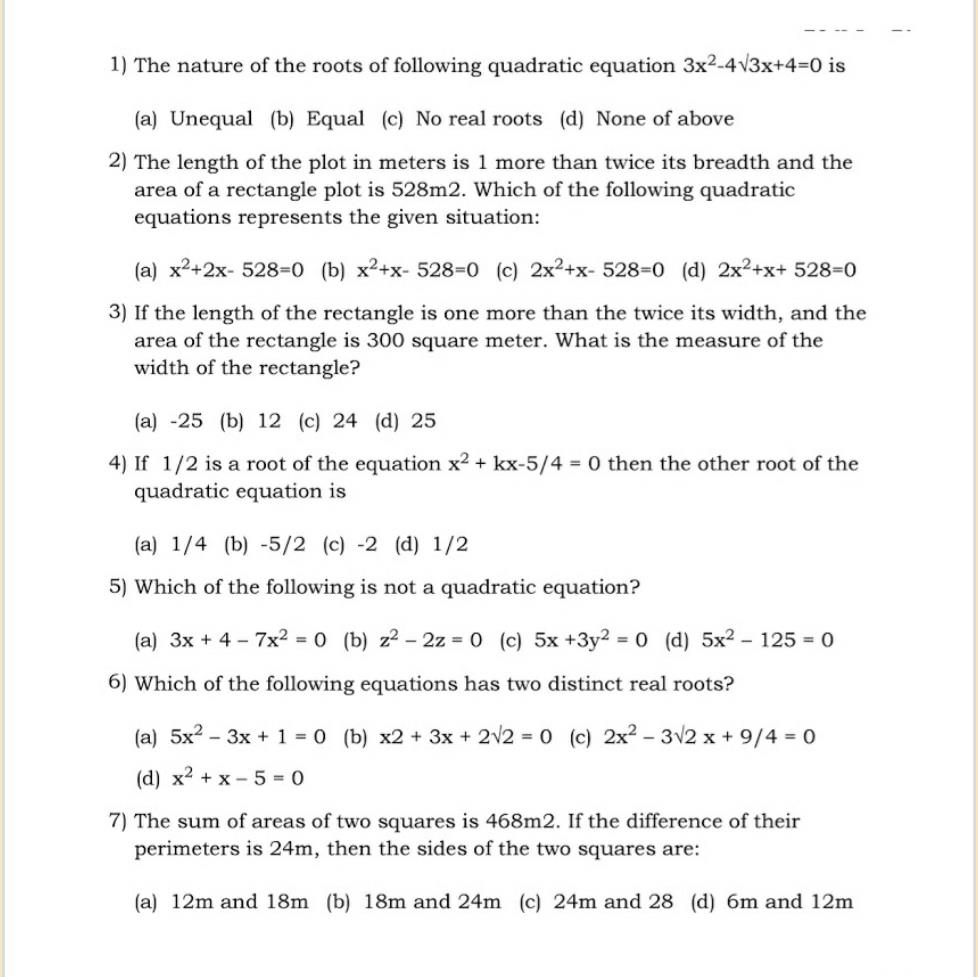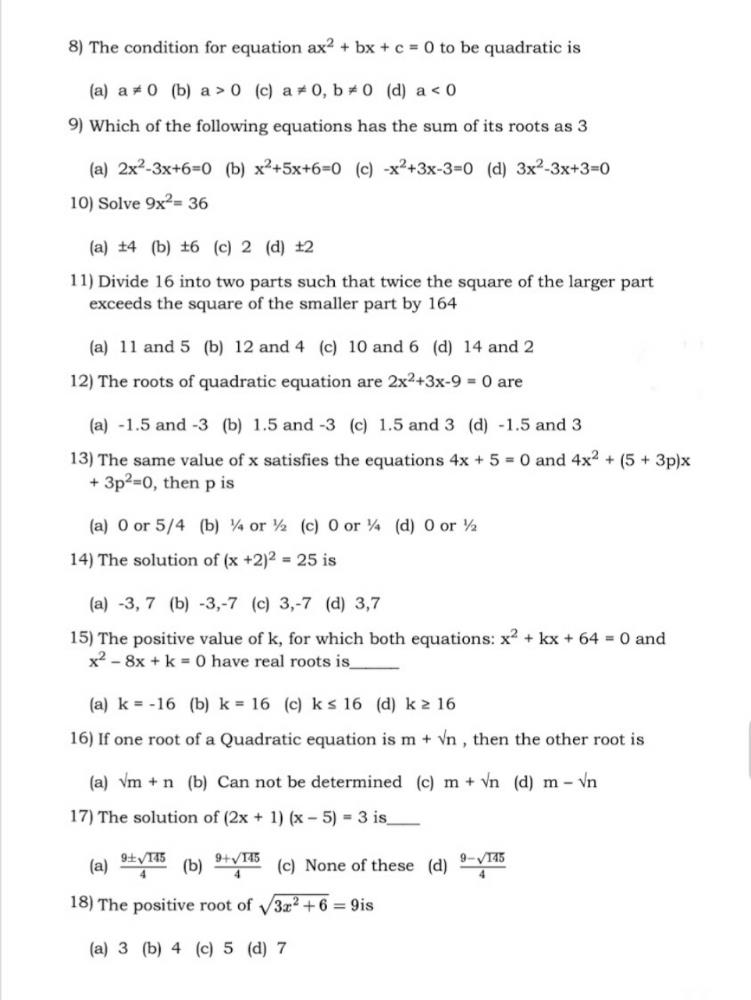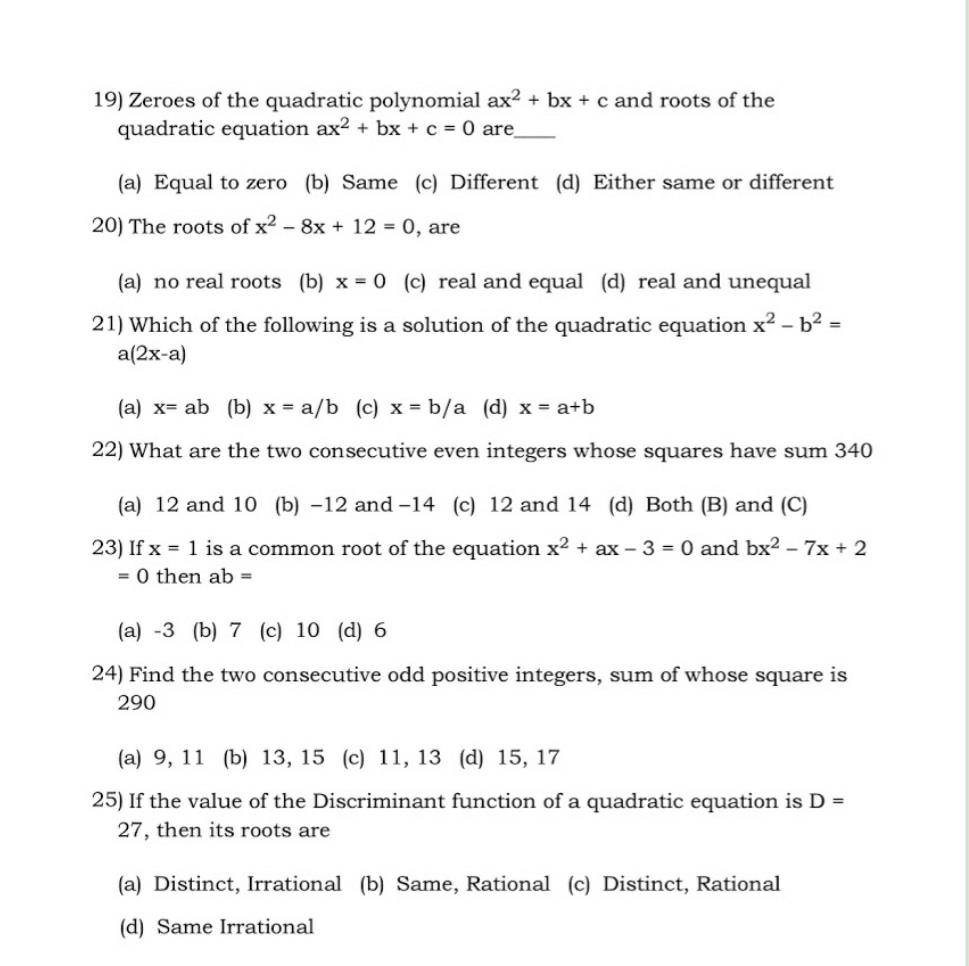Courses

Math: Quadratic equations: Important One mark Questions--Class 10 Notes - Class 10

Class 10: Math: Quadratic equations: Important One mark Questions--Class 10 Notes - Class 10

The document Math: Quadratic equations: Important One mark Questions--Class 10 Notes - Class 10 is a part of Class 10 category.
All you need of Class 10 at this link: Class 10The Document consists of 25 questions which were repeatedly asked in the tests and Exams.
Practise these problems.Here are some formulaes which could be helpful for you guys:

1. Formula for Verifying the Zeroes of the quadratic polynomial;
α β = -b / a
α×β = c/a
2.Area of a Rectangle = length × breadth
3.Area of a Square = Side × Side
4.Perimeter of a square = 4 × side
5.Consecutive Even integers = X, X 2
6.Consecutie odd positive integers = X, X 2
7.If the discriminant is less than 0,then the nature of roots will be NO REAL ROOTS.
8.If the discriminant is equal to 0,then the nature of roots will be REAL AND EQUAL ROOTS.
9.If the discriminant is more than 0,then the nature of roots will be REAL AND DISTINCT ROOTS.

The document Math: Quadratic equations: Important One mark Questions--Class 10 Notes - Class 10 is a part of Class 10 category.
All you need of Class 10 at this link: Class 10Use Code STAYHOME200 and get INR 200 additional OFF Use Coupon Code

Top Courses for Class 10Top Courses for Class 10Track your progress, build streaks, highlight & save important lessons and more!

,

,

,

,

,

,

,

,

,

,

,

,

,

,

,

,

,

,

,

,

,

;